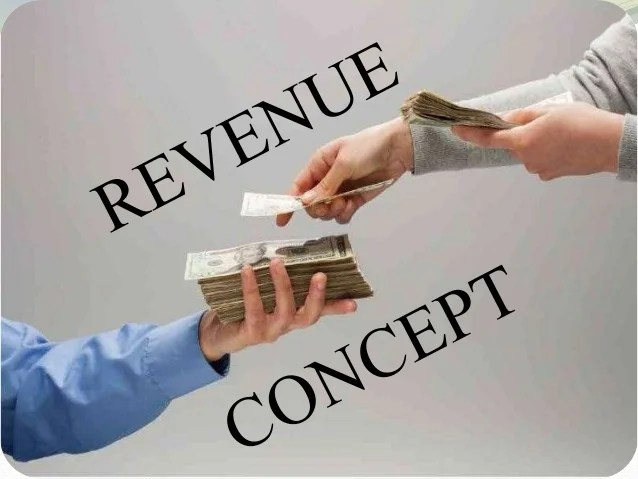# Revenue Concept

Welcome to class!

In today’s class, we will be talking about revenue concept. Enjoy the class!

### Revenue Concept#### The meaning of revenue to an economist

The term revenue refers to the money obtained by a firm through the sale of goods at different prices and over a certain period of time. In other words, revenue is the total amount of money that a company actually receives for selling its products and services during a specific period of time (like a year). This total income includes all the discounts and deductions for all merchandise. To put it in the purest economic terms, it is the “top line” or “gross income” figure from which costs are subtracted to determine net income.

##### Types of revenue

There are three types of revenue you should know, and these include-

1. Total Revenue
2. Average Revenue
3. Marginal Revenue
• Total Revenue: This is the total receipts from the sales of a given quantity of goods/services. It is exactly what its name suggests- the total income of a business. To calculate the total income, multiply the quantity of goods sold by the price of the goods. You can also calculate it as the selling price of a firm’s product times the quantity sold (i.e. total revenue = price × quantity). You can also let Total Report be the total revenue function: TR(Q)=P(Q) X Q

such “Q ” is the quantity of output sold and “P(Q)” is the inverse demand function.

• Average Revenue: Average revenue is the revenue generated per unit of output sold. It plays a role in the determination of a firm’s profit. Per unit profit is average revenue minus average (total) cost. A firm generally seeks to produce the quantity of output that maximizes profit.
• Marginal Revenue: Marginal Revenue is the additional revenue that will be generated by increasing product sales by one unit. It can also be described as the unit revenue the last item sold has generated for the firm. As a result, it will have to lower the price of all units sold to increase sales by 1 unit.

Marginal revenue is equal to the ratio of the change in revenue for some change in quantity sold to that change in quantity sold. This can also be represented as a derivative when the change in quantity sold becomes arbitrarily small. More formally, define the revenue function to be the following

R(q)=P(q) . q

By the product rule, marginal revenue is then given by

R'(q)=P(q)+P'(q) . q.

For a firm facing perfect competition, price does not change with quantity sold

P'(q)=0, so marginal revenue is equal to price. For a monopoly, the price decreases with quantity sold P'(q)<0, so marginal revenue is less than price (for q).

Typical marginal revenue R’ and average revenue (price) <R> curves for a firm that is not in perfect competition.In our next class, we will be talking about the Economic Concept.  We hope you enjoyed the class.

Should you have any further question, feel free to ask in the comment section below and trust us to respond as soon as possible.